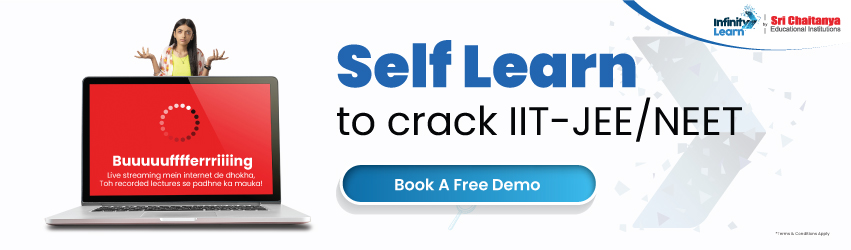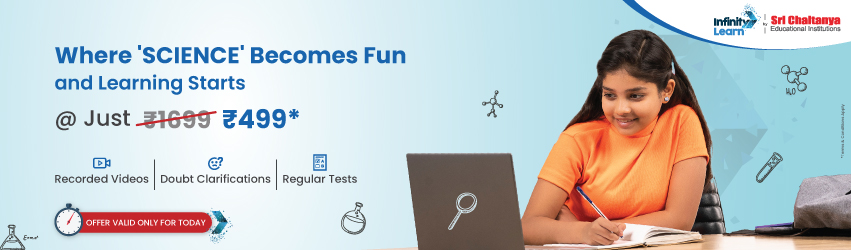RD Sharma Class 11 Solutions Chapter 32 Statistics Ex 32.5

# RD Sharma Class 11 Solutions Chapter 32 Statistics Ex 32.5infinitylearn surge self learn
infinitylearn starter package

## RD Sharma Class 11 Solutions Chapter 32 Statistics Ex 32.5

• Chapter 32 Statistics Ex 32.1
• Chapter 32 Statistics Ex 32.2
• Chapter 32 Statistics Ex 32.3
• Chapter 32 Statistics Ex 32.4
• Chapter 32 Statistics Ex 32.5
• Chapter 32 Statistics Ex 32.6
• Chapter 32 Statistics Ex 32.7

Statistics Ex 32.5 Q1Statistics Ex 32.5 Q2Statistics Ex 32.5 Q3Statistics Ex 32.5 Q4Need FREE NCERT/CBSE/IIT-JEE/NEET Study Material?